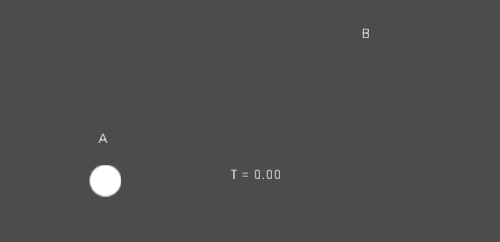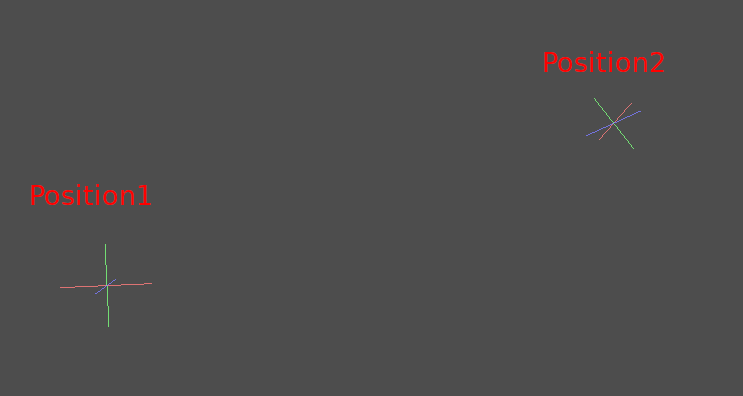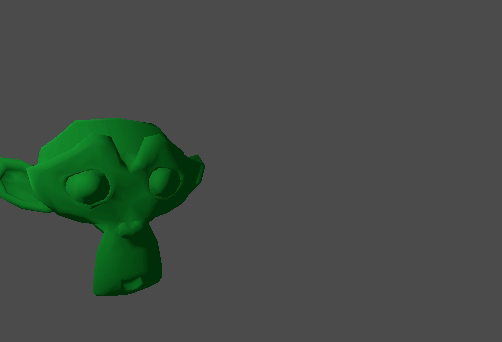# Interpolation¶

Interpolation is a very basic operation in graphics programming. It’s good to become familiar with it in order to expand your horizons as a graphics developer.

The basic idea is that you want to transition from A to B. A value `t`, represents the states in-between.

As an example if `t` is 0, then the state is A. If `t` is 1, then the state is B. Anything in-between is an interpolation.

Between two real (floating point) numbers, a simple interpolation is usually described as:

```interpolation = A * (1 - t) + B * t
```

And often simplified to:

```interpolation = A + (B - A) * t
```

The name of this type of interpolation, which transforms a value into another at constant speed is «linear». So, when you hear about Linear Interpolation, you know they are referring to this simple formula.

There are other types of interpolations, which will not be covered here. A recommended read afterwards is the Bezier page.

## Vector interpolation¶

Vector types (Vector2 and Vector3) can also be interpolated, they come with handy functions to do it Vector2.linear_interpolate() and Vector3.linear_interpolate().

For cubic interpolation, there are also Vector2.cubic_interpolate() and Vector3.cubic_interpolate(), which do a Bezier style interpolation.

Here is simple pseudo-code for going from point A to B using interpolation:

```func _physics_process(delta):
t += delta * 0.4

\$Sprite.position = \$A.position.linear_interpolate(\$B.position, t)
```

It will produce the following motion:## Transform interpolation¶

It is also possible to interpolate whole transforms (make sure they have either uniform scale or, at least, the same non-uniform scale). For this, the function Transform.interpolate_with() can be used.

Here is an example of transforming a monkey from Position1 to Position2:Using the following pseudocode:

```var t = 0.0

func _physics_process(delta):
t += delta

\$Monkey.transform = \$Position1.transform.interpolate_with(\$Position2.transform, t)
```

And again, it will produce the following motion:## Smoothing motion¶

Interpolation can be used to smooth movement, rotation, etc. Here is an example of a circle following the mouse using smoothed motion:

```const FOLLOW_SPEED = 4.0

func _physics_process(delta):
var mouse_pos = get_local_mouse_position()

\$Sprite.position = \$Sprite.position.linear_interpolate(mouse_pos, delta * FOLLOW_SPEED)
```

Here is how it looks:This useful for smoothing camera movement, allies following you (ensuring they stay within a certain range), and many other common game patterns.